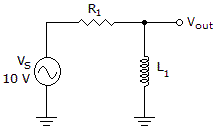# Electronics - RL Circuits

### Exercise :: RL Circuits - General Questions

11.Which of the following statements is true if R = 100and XL = 100in the circuit in the given circuit?

 A. Each component drops 5 V. B. The impedance equals 200. C. The power factor equals 1. D. The phase angle equals 45°.

Explanation:

No answer description available for this question. Let us discuss.

12.

Which of the following statements is true about a lag network?

 A. An increase in frequency causes an increase in phase lag. B. An increase in frequency causes an increase in the magnitude of the output voltage. C. A decrease in frequency causes an increase in phase lag. D. A decrease in frequency causes a decrease in the magnitude of the output voltage.